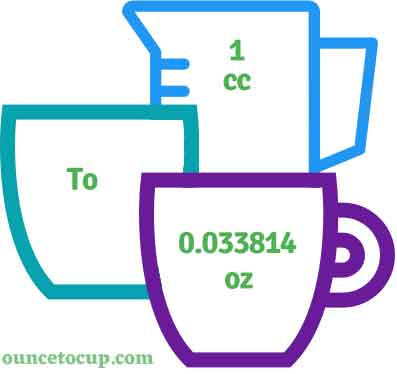# Cubic Centimeter to Ounce (cc to oz conversion)

Are you cooking your favorite dish? The detailed chart in the recipe includes the calculation of the 1 cubic centimeter to ounce conversion.  Don't worry; use this calculator to determine how many 1 cubic centimeter equals ounces in a minute.  This 1 cm^3 to oz converter gives an exact measurement for any recipe you prepare.

Cubic Centimeter Value:

cc

Ounce Value:

oz

1 Cubic Centimeter = 0.033814 Ounce
(1 cc = 0.033814 oz)

Try our auto 1 Cubic Centimeter to Ounce Calculator (Without Convert Button), Just change the first field value and you got final value.## How many oz is a cc?

We know that the volume value of 1 cm^3 is equal to 0.033814 oz. If you want to convert 1 fluid cc to an equal number of oz, just multiply the volume value by 0.033814 or divide the value by 29.5735. Hence, 1 cubic centimeter is equal to 0.033814 oz.

The Answer is: 1 US Fluid Cubic Centimeters = 0.033814 US Ounce

1 cc = 0.033814 oz

Many of them try to search or find an answer for what is 1 cubic centimeters in oz? So, we’ll start with 1 cm^3 to oz conversion to know how big is 1 cc.

## How To Calculate 1 fluid cc to oz?

To calculate 1 fluid cubic centimeters to an equal number of ounce, simply follow the steps below.

Fluid Cubic Centimeters to Ounces formula is:

Ounce = Fluid Cubic Centimeter * 0.033814

Assume that we are finding out how many oz were found in 1 fl cc of water, multiply by 0.033814 to get the result.

Applying to Formula: oz = 1 cc * 0.033814 = 0.033814 oz.

## How To Convert cc to oz?

• To convert fluid cubic centimeters to oz,
• Simply multiply the fluid cubic centimeter value by 0.033814 (or) simply divide them by 29.5735.
• Applying to the formula, oz = 1 cubic centimeters * 0.033814 [1x0.033814].
• Hence, 1 cubic centimeters is equal to 0.033814 oz.

## Some quick table references for cubic centimeter to ounce conversions:

Cubic Centimeter [cc or cm^3]Ounces [oz]
1 cc0.0338 oz
2 cc0.0676 oz
3 cc0.1014 oz
4 cc0.1353 oz
5 cc0.1691 oz
6 cc0.2029 oz
7 cc0.2367 oz
8 cc0.2705 oz
9 cc0.3043 oz
10 cc0.3381 oz
11 cc0.372 oz
12 cc0.4058 oz
13 cc0.4396 oz
14 cc0.4734 oz
15 cc0.5072 oz

## Reverse Calculation: How many cubic centimeters are in a oz?

• To convert 1 oz to cc,
• Simply multiply the 1 oz by 29.5735.
• Then, applying the formula, cc = 1 oz * 29.5735 [1x29.5735 = 29.5735].
• Hence, 1 oz is equal to 29.5735 cc.

### Related Converter:

Formula: cubic centimeter to oz

oz = cubic centimeter * 0.033814

Applying to Formula,

oz = 1*0.033814 = 0.033814

1 cc = 0.033814 oz